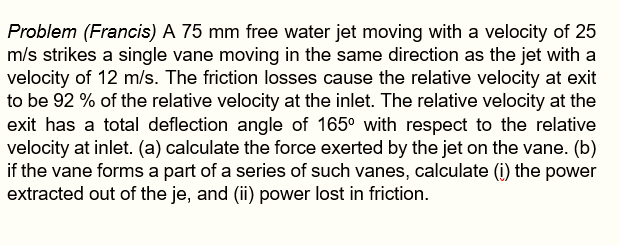# Question Solved1 AnswerProblem (Francis) A 75 mm free water jet moving with a velocity of 25 m/s strikes a single vane moving in the same direction as the jet with a velocity of 12 m/s. The friction losses cause the relative velocity at exit to be 92 % of the relative velocity at the inlet. The relative velocity at the exit has a total deflection angle of 165° with respect to the relative velocity at inlet. (a) calculate the force exerted by the jet on the vane. (b) if the vane forms a part of a series of such vanes, calculate (i) the power extracted out of the je, and (ii) power lost in friction.K64ALA The Asker · Mechanical EngineeringTranscribed Image Text: Problem (Francis) A 75 mm free water jet moving with a velocity of 25 m/s strikes a single vane moving in the same direction as the jet with a velocity of 12 m/s. The friction losses cause the relative velocity at exit to be 92 % of the relative velocity at the inlet. The relative velocity at the exit has a total deflection angle of 165° with respect to the relative velocity at inlet. (a) calculate the force exerted by the jet on the vane. (b) if the vane forms a part of a series of such vanes, calculate (i) the power extracted out of the je, and (ii) power lost in friction.
More
Transcribed Image Text: Problem (Francis) A 75 mm free water jet moving with a velocity of 25 m/s strikes a single vane moving in the same direction as the jet with a velocity of 12 m/s. The friction losses cause the relative velocity at exit to be 92 % of the relative velocity at the inlet. The relative velocity at the exit has a total deflection angle of 165° with respect to the relative velocity at inlet. (a) calculate the force exerted by the jet on the vane. (b) if the vane forms a part of a series of such vanes, calculate (i) the power extracted out of the je, and (ii) power lost in friction.